# Set size + position on drag

#1

Hi Forum, I have the following situation: A slider that is used to set a price filter.

I would like to set up the following interaction:
Starting state: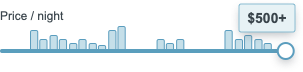End state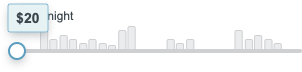Currently I have set up the Dragged functionality as follows:

Case Price: Will be duplicated to set the text reflected in the input+the value of the global variable when the ‘trigger’ is over the area of that value on the slider.
Case Slider: moving the trigger within the boundaries along the slider and setting the size of the blue bar + blue tooltip background

CHALLENGE 1: The boundary is currently not working as desired on the left side. As you can see it goes out of the boundary, which should be the left side of the trigger. Basically resulting in the tooltip shifting from right aligned at start to left aligned at finish on the far left.

CHALLENGE 2: The blue tooltip background is currently not changing the size, even though I have set up a set size action. I would like it to set the size based on the value of the variable + currency.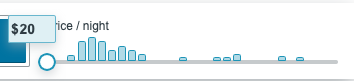File attachment:
Filters_PriceSlider.rp (61.2 KB)

#2

Hi!

The reason Trigger is going outside of the bounds is because you are setting its left limit to the left side of the bar minus half the width of Trigger. Get rid of the minus part if you want the left side of trigger to be left aligned with Bar. So left limit should be greater than or equal to [[Bar.left]] and the right limit should be less than or equal to [[Bar.right]]

The second one is more difficult. First, rather than using the text of currency plus the text of price (which results to “not a number” since “\$” is not a number), it seems like you want to be adding the width of those widgets together. E.g., put the widget of each widget in local variables (rather than the text of each), and then say [[price.width + currency.width]].

However, the bad news is that Axure does not increase the width of a widget when you increase the width of its text. If you have a widget that is 10px wide, and then you set its text to “XXXXXXXXXXXXXXXXXXXX” the widget will remain 10px wide. There is no way to get the width of a text string in Axure without using javascript.

You’ll probably want to hack the width setting by using which hotspot Trigger is above.

Or, since numbers in many fonts are fixed width, you can use the width of a single character and multiply it by how many characters there are. To get how many characters are in a widget’s text, set the local variable to the widgets text and then use [[LVAR.length]].

#3

Hi,

Challenge 2:

Are you referring to applying the set size action in the Case - Price?
Just writing it out: If the area of the trigger is over a hotspot, set the tooltip value to ##, then set the size to tooltip value width?

This one seemed like a simpler solution and seems to work.

Challenge 1:
The issue is with the tooltip (rather than the trigger) not being left aligned. I’d like the end state to be this:but its currently like this at the moment: Firstly with the resize it does not align to the right anymore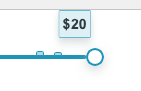Secondly the tooltip still goes over the boundary on the left side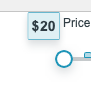#4

Hi!

When dealing with a slider, I find it’s useful use an expression that gives you the current value of the slider. Then you can use that number to calculate a bunch of different of things: the x location of the tooltip, for example, but also the price for the given slider value.

A useful output value for the slider is 0 when at the far left and 1 when at the far right. So if the slider is halfway, this value would be 0.5.

You can calculate this by dividing the x location of the slider handle (your Trigger widget) by the maximum distance the slider handle can travel. That distance is the width of the slider minus the width of the handle, assuming the handle stops at each end of the slider. So…

``````set text on v_sliderVal to [[ Target.x / (slider.width - Target.width) ]]
``````

…where `Target` is the handle and `slider` is a local variable referring to the slider (here, your “grey bar”). Note that this assumes that the grey bar is placed at x=0 in the dynamic panel.

So let’s assume the slider is 1050px wide and the handle is 50px wide. The maximum travel is then 1000px:

• If the slider handle is at x=0, or all the way left, then 0 / 1000 = 0
• If the slider handle is at x=1000, or all the way right, then 1000 / 1000 = 1
• If the slider handle is at x=500, or in the middle, then 500 / 1000 = 0.5

Now you can use this number to place the tooltip. The maximum distance the tooltip can travel (if you want to keep it within the bounds of the slider) is the width of the slider minus the width of the tooltip. So assuming `sliderVal` is this 0-to-1 value that you’ve already calculated, and `slider` and `tooltip` are local variables referring to those two widgets:

``````Move tooltip to [[sliderVal * (slider.width - tooltip.width) ]]
``````

Likewise, you can use the slider value to calculate the price. Assuming `min` is the minimum price and `max` is the maximum price, and `sliderVal` is this 0-to-1 number:

``````Set text on price to [[ min + sliderVal * (max - min) ]]
``````

Since you don’t want an additional 10 digits after the decimal, you can use a function to round it:

``````[[ (min + sliderVal * (max - min)).toFixed(0) ]]
``````

I updated your file. I also added the ability to round to the nearest unit, in this case, the nearest 10. You can set this to 1 if you want it to the nearest 1. The explanation of that math is here.

Note: I also changed the left and right limits of your drag command. They now use greater than/less than or equals

Preview

File: Filters_PriceSlider.rp (85.1 KB)

#5

Hi josephxbrick, ok there is some crazy maths happening here. Thank you so much for that.

Of course by solving these two challenges I am no facing 1 (or 2) more.

So I’ve managed to get your function working, plus a solution to resize the tooltip.

BUT

The value+currency does not remain in the centre of the tooltip background, and the tooltip no longer remains right aligned to the handle.

Is there a way I can ensure the currency+value always remain in the centre, and the tooltip background remains actually right aligned after resizing at \$600+? You’ll notice in the preview that there is a weird jump at the end which actually aligns the tooltip background to the centre of the handle instead of the right of the handle.

Filters_PriceSlider.rp (59.8 KB)

#6

Hi!

A lot of the issues go away if we make one basic change. Make the tooltip simply a rectangle and set the text of the rectangle, adding the currency symbol onto the text string. A nice side effect is that this will automatically center the text within the rectangle, since you can set the text alignment of the rectangle to center.

The reason for the tooltip jumping at the end of the slider is the anchoring of the resize command. (The anchor is the edge that sizing resizes out from.) It’s resizing from the left, which is fine when the tooltip is in the left half of the slider, but when the tooltip is in the right half of the slider, it’s a problem. You want to set the anchoring at left when the tooltip is left of center, and set the anchoring at right when the tooltip is right of center.

Since there is so much going on with the tooltip, I created a “function” to update the tooltip’s text, size and location, rather than having all of that functionality in the middle of the Dragged event.

Axure doesn’t have functions, so the common trick is to create an external “function” widget (I use a tooltip), and when you want to execute the function (e.g., from the Dragged event), move the tooltip by 0,0. The tooltip then handles the Moved event that is triggered by the Move command, and runs the On Moved code. I always name function widgets starting with “function_” so I can tell what they’re for.

The function does the following:

• Sets the text of the tooltip appropriately (including the currency)
• Adds the plus if sliderVal equals 1 (meaning all the way to the right)
• If sliderVal is less than 0.5, meaning the handle is in the left half of the slider, size the tooltip with the anchor at the left; else, use the size command with the anchor at the right
• Move the tooltip to the proper spot along the slider.

Note that the function hotspot’s On Loaded event moves the hotspot, which runs the function. This initializes the tooltip when the page loads.

If you want to change the expression that resizes the tooltip, make sure you change it in both Size commands (the one anchoring from the left and the one anchoring from the right.)

Note: do not use animation when resizing the tooltip, as Axure 9 has a bug when using animation within the dragged event. Suddenly the thing you are dragging moves to the wrong location entirely. The latest Axure update was supposed to fix this well known issue, but it’s still broken. I will report this to the Axure folks.

Here is the updated file: Filters_PriceSlider (1).rp (62.1 KB)

#7

Hi! That’s some really neat work to creating a function! Very insightful! It works 99.9% perfectly.

I’ve just added the following function, in order to be able to update the global variable so that it can be used to actually apply the pricevalue as a filter to a repeater.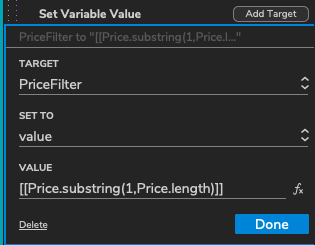Thanks a lot for your huge input with the whole thing!

#8

Hi, I have one more question actually;

Is there a way I can use the sliderVal to disable or set the style of the nightly-bars so that any of the bars which fall to the right of the handle are disabled/greyed out.

Something like this: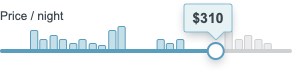Thank you!

#9

Hi!

Yes. You can use this “function” trick I mentioned so that each bar checks itself to see if it should light up or not.

You’d add code like the code below to each bar. The code will be exactly the same for each bar, so once you get the Moved event it working for one bar, you can just copy/paste the whole Moved event (containing both conditions) to all bars. Or, you can just delete all of the other bars, and then copy/paste the working bar over and over…

Note that this assumes that you are using the bar’s Disabled style effect to make the bar inactive.

``````On Moved (of a bar: this is the "function" you will call by moving this bar by 0,0)
If value [[LVAR_handle.left + LVAR_handle.width/2]] is greater than or equals value [[This.left + this.width/2]]
Set enabled of this to true
else if
Set disabled of this to false
``````

The code assumes that the bar lights up when the center of the slider is at or to the right of the center of the bar. That’s what the `+this.width/2` part is is for. (The left of something plus half its width gives you the center of that something.)

Then, important! In the handle’s Dragged event, move all of the bars by 0,0.

I set this up on the first set of bars.

Filters_PriceSlider (1).rp (70.0 KB)

#10

Hi, amazing! Thanks so much for the inputIt works perfectly now